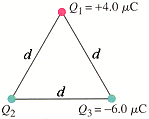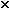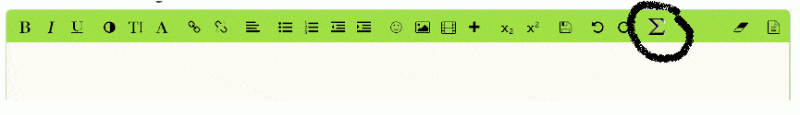# Some Electrostatics Help?

## Homework Statement

The total electric flux from a cubical box 34.0 cm on a side is 1.29 x 103 N·m2/C. What charge is enclosed by the box?

----and----

Three charged particles are placed at the corners of an equilateral triangle of side d = 1.00 m (Fig. 16-53). The charges are Q1 = +4.0 µC, Q2 = -6.0µC, and Q3 = -6.0 µC. Calculate the magnitude and direction of the net force on each due to the other two.Figure 16-53
Force on Q1: N at ° counterclockwise from +x axis (to the right)
Force on Q2: N at ° counterclockwise from +x axis (to the right)
Force on Q3: N at ° counterclockwise from +x axis (to the right)

## Homework Equations

Gauss' Law (I think)(I don't know how to type those symbols out sooo)

----and----

I'm not really sure on this one. I've tried setting it up F=(k)(d^2/|Q(#)Q(#)) to find the magnitude but that isn't working (or at least my numbers are weird for it)

## The Attempt at a Solution

I tried to multiply (1.29103 N·m2/C)(8.85e-12) but that didn't work? Do I (and if it is needed) how do I incorporate the 34cm?

----and----

See above

Last edited by a moderator:

## Answers and Replies

gneill
Mentor
One question per post please.

Can you show some actual calculation results? "that didn't work" or "that isn't working" doesn't tell us much.

You can find most of the symbols you need by selecting the Sigma icon from the editing menu bar: